# PSEB 5th Class Maths Solutions Chapter 6 Measurement Ex 6.1

Punjab State Board PSEB 5th Class Maths Book Solutions Chapter 6 Measurement Ex 6.1 Textbook Exercise Questions and Answers.

## PSEB Solutions for Class 5 Maths Chapter 6 Measurement Ex 6.1

1. Find the length:

Question 1.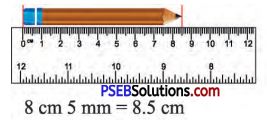Question 2.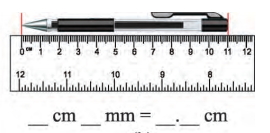Solution:
11 cm 0 cm = 11 cmQuestion 3.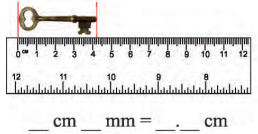Solution:
4 cm 2 mm = 4.2 cm

Question 4.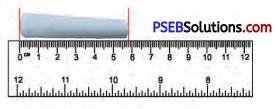Solution:
5 cm 8 mm = 5.8 cm

2. Measure the length of line segments in cm and draw on your notebooks.

Question 1.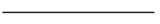Solution:
3.6 cmQuestion 2.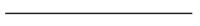Solution:
4.4 cmQuestion 3.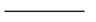Solution:
1.9 cmQuestion 4.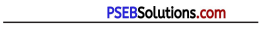Solution:
6 cmQuestion 5.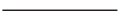Solution:
2.7 cmQuestion 6.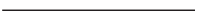Solution:
4.5 cm3. Fill in the blanks:

Question 1.
3.45 m = …………….. m ……………….. cm
Solution:
3 m 45 cm

Question 2.
5.75 m = ……………. m ……………. cm
Solution:
5 m 75 cm

Question 3.
10.850 km = ………………. km …………….. m
Solution:
10 km 850 mQuestion 4.
………………….. m = 4 m 25 cm
Solution:
4.25 m

Question 5.
……………… km = 7 km 375 m
Solution:
7.375 km

4. Convert the following:

Question 1.
4.5 cm into mm
Solution:
4.5 cm = 4.5 × 10 mm = 45 mm

Question 2.
270 m into km
Solution:
270 m = $$\frac{270}{1000}$$ km = 0.270 km

Question 3.
5.82 km into m
Solution:
5.82 = 5.82 × 1000 m = 5820 mQuestion 4.
0.65 m into cm
Solution:
0.65 = 0.65 × 100 cm = 65 cm

Question 5.
18 mm into m
Solution:
18 mm = $$\frac{18}{1000}$$ m = 0.018 m